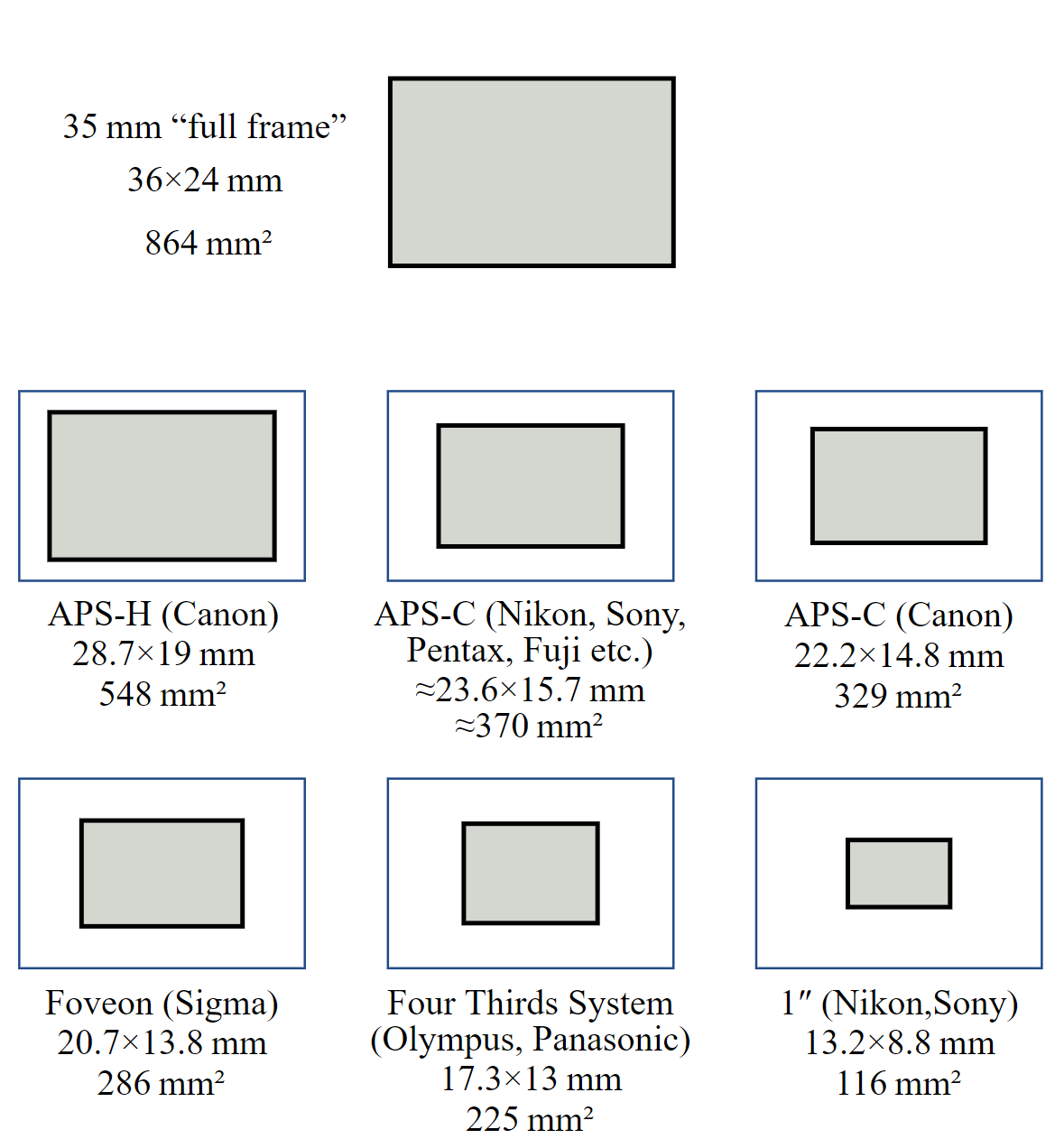0%$残画幅焦距 = \frac{全画幅焦距}{裁切系数}$

$残画幅光圈值 = \frac{全画幅光圈值}{裁切系数}$

$残画幅感光度 = \frac{全画幅感光度}{裁切系数^2}$